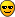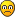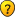## Sudoku Symmetry Group (minimal spec)

Everything about Sudoku that doesn't fit in one of the other sections

### Re: Sudoku Symmetry Group (minimal spec)

blue wrote:How come his feet aren't where his head should be, and vica-versa ?

His feet could be in his mouth, like mine usually areMathimagics
2017 Supporter

Posts: 1481
Joined: 27 May 2015
Location: Canberra

### Re: Sudoku Symmetry Group (minimal spec)

deleted.
Last edited by StrmCkr on Sun Dec 23, 2018 9:05 am, edited 1 time in total.
Some do, some teach, the rest look it up.StrmCkr

Posts: 1085
Joined: 05 September 2006

### Proofiness

.
Some observations that might be useful in the search for proofiness (not to be confused with "truthiness").

Consider the case of simple Latin Squares of order N (note, not N^2). The order of the symmetry group LG(N) is (N! ^ 2 * 2).

Now that we have one less "dimension" (no bands), it is not surprising that we find these groups can be generated by just 2 permutations {x, y}.

This SG2-style generator seems to do the job (although note that it is sensitive to the parity of N):

• x = ShiftR(N-1) for odd N
• x = ShiftR(N-2) for even N
• y = Rotate 90

Interestingly, I can't seem to find an equivalent SG1-style generator, ie. any {x, y} with y = flip.

Perhaps this group might be easier to analyse? If so, it might lead us to a method for solving the SG proof problem.Mathimagics
2017 Supporter

Posts: 1481
Joined: 27 May 2015
Location: Canberra

### Re: Sudoku Symmetry Group (minimal spec)

deleted.
Last edited by StrmCkr on Sun Dec 23, 2018 9:10 am, edited 1 time in total.
Some do, some teach, the rest look it up.StrmCkr

Posts: 1085
Joined: 05 September 2006

### Re: Sudoku Symmetry Group (minimal spec)

deleted
Last edited by blue on Sun Dec 23, 2018 10:32 am, edited 1 time in total.
blue

Posts: 894
Joined: 11 March 2013

### Re: Sudoku Symmetry Group (minimal spec)

deleted
Last edited by StrmCkr on Sun Dec 23, 2018 9:05 am, edited 1 time in total.
Some do, some teach, the rest look it up.StrmCkr

Posts: 1085
Joined: 05 September 2006

### Re: Sudoku Symmetry Group (minimal spec)

deleted
Last edited by blue on Sun Dec 23, 2018 10:32 am, edited 1 time in total.
blue

Posts: 894
Joined: 11 March 2013

### Re: Proofiness

Mathimagics wrote:.
Some observations that might be useful in the search for proofiness (not to be confused with "truthiness").

Consider the case of simple Latin Squares of order N (note, not N^2). The order of the symmetry group LG(N) is (N! ^ 2 * 2).

Now that we have one less "dimension" (no bands), it is not surprising that we find these groups can be generated by just 2 permutations {x, y}.

This SG2-style generator seems to do the job (although note that it is sensitive to the parity of N):

• x = ShiftR(N-1) for odd N
• x = ShiftR(N-2) for even N
• y = Rotate 90

Interestingly, I can't seem to find an equivalent SG1-style generator, ie. any {x, y} with y = flip.

That seems to work for all odd N.
For even N, it looks like it only works for N >= 8.
(For N=2, ShiftR(0) doesn't make sense ... ShiftR(1) is the "no nothing" operation).
Checked with GAP, for N=3,4,...,40.

This, seems to work for all N >= 2 ... checked for N=2,...,40:

• x = ShiftR(N) + ShiftC(N-1)
• y = Flip
blue

Posts: 894
Joined: 11 March 2013

### Re: Proofiness

For Latin Squares:

blue wrote:This, seems to work for all N >= 2 ... checked for N=2,...,40:

• x = ShiftR(N) + ShiftC(N-1)
• y = Flip

Similarly, for Sudoku, this seems to work:

• x = ShiftR(N) + ShiftC(N-1)
• y = ShiftB(N) + ShiftS(N-1)
• z = Flip
Checked for N=2,...,10 with GAP.

Added: It's easy to prove that these work for all N, too.
Last edited by blue on Sat Dec 22, 2018 9:33 pm, edited 1 time in total.
blue

Posts: 894
Joined: 11 March 2013

### Re: Sudoku Symmetry Group (minimal spec)

.
Ok, that's nice! We'll add that to the catalog ...

I'll have to revise my formulation, I thought I had checked N=6 but obviously not!blue wrote:For N=2, ShiftR(0) doesn't make sense ...

Unless you accept that Shift(0) = Shift(1) = ()Mathimagics
2017 Supporter

Posts: 1481
Joined: 27 May 2015
Location: Canberra

### Re: Sudoku Symmetry Group (minimal spec)

Mathimagics wrote:Unless you accept that Shift(0) = Shift(1) = ()

True.
It's just my GAP code that breaks down.Code: Select all
`gap> cycle := function(N,n) return Concatenation([2..n],,[n+1..N]); end;;gap> cycle(3,1);[ 1, 2, 3 ]gap> cycle(3,2);[ 2, 1, 3 ]gap> cycle(3,3);[ 2, 3, 1 ]gap> cycle(3,0);[ 1, 1, 2, 3 ]gap> PermList(cycle(3,1));()gap> PermList(cycle(3,2));(1,2)gap> PermList(cycle(3,3));(1,2,3)gap> PermList(cycle(3,0));failgap>`
blue

Posts: 894
Joined: 11 March 2013

### Re: Sudoku Symmetry Group (minimal spec)

.
Try this:
Code: Select all
`cycle := function(N,n) if (n < 2) then n  := 1; fi; return Concatenation([2..n],,[n+1..N]); end;;`Mathimagics
2017 Supporter

Posts: 1481
Joined: 27 May 2015
Location: Canberra

### Re: Sudoku Symmetry Group (minimal spec)

Transpose (aka flip) IS a diagonal reflection (main diagonal).

Rotate quarter turn is different.

well, apparently i didn't see this correction point earlier i would have dropped everything from the get go....

.......that's what i get for 2 much work and no sleep... thanks for bearing with me....

and
spelled right out in
blues post
None of the individual operations are quarter turns, but ...
"1 -> 2 -> 3" gives a CW quarter turn (overall), and "2 -> 3 -> 4" gives a CCW quarter turn.

i clearly missed the 1/4 turn reference again in blues sub point that diagonal reflection + vertical or horizontal reflection = 90 CCW rotation or 90 Cw rotation.

the good thing is Ive now put (1/4 ) turn back-into my solver since it was missing from the rebuild.
and i learned there is a reference to which is the clearly called the "main diagonal"
Some do, some teach, the rest look it up.StrmCkr

Posts: 1085
Joined: 05 September 2006

### Re: Proofiness

blue wrote:Similarly, for Sudoku, this seems to work:

• x = ShiftR(N) + ShiftC(N-1)
• y = ShiftB(N) + ShiftS(N-1)
• z = Flip
Checked for N=2,...,10 with GAP.

Added: It's easy to prove that these work for all N, too.

Anything provable is quite exciting!Can we see the proof, or an outline thereof[EDIT] LS generators verified to N = 70, SG generators to N = 11 (!). N = 12 crashes Gap on my Win64 system, so that's my absolute limit.
As for my rotation-based SG generator, it's failure on just N=4, N=6 is really intriguing. Clearly something to do with rotate quarter-turn failing to combine with the other x permutation in enough ways. Dihedral group properties? I'm looking into the corresponding Cayley tables to see if there is an explanation.Mathimagics
2017 Supporter

Posts: 1481
Joined: 27 May 2015
Location: Canberra

### Re: Proofiness

Starting from:

• x = ShiftR(N) + ShiftC(N-1)
• y = ShiftB(N) + ShiftS(N-1)
• z = Flip
The factors in x commute, and we have:
x^k = ShiftR(N)^k * ShiftC(N-1)^k
With that we can derive ...

x^(N-1)
= ShiftR(N)^(N-1) * ShiftC(N-1)^(N-1)
= ShiftR(N)^(-1) * ShiftR(N)^N * ShiftC(N-1)^(N-1)
= ShiftR(N)^(-1)
x^N
= ShiftR(N)^N * ShiftC(N-1)^N
= ShiftR(N)^N * ShiftC(N-1)^(N-1) * ShiftC(N-1)
= ShiftC(N-1)
x^(N*(N-1)) = 1

z*x^N*z
= Flip + ShiftC(N-1) + Flip
= ShiftR(N-1)
Using 'y' instead of 'x', we get similar results for ShiftB()

Summarizing, we have
Code: Select all
`1) Flip = z2) ShiftR(N)^(-1) = x^(N-1)3) ShiftR(N-1)    = z*x^N*z4) ShiftB(N)^(-1) = y^(N-1)5) ShiftB(N-1)    = z*y^N*z`

And if we like:
Code: Select all
`6) ShiftR(N) = x^((N-1)*(N-1)) ... from (2) and (x^(N*(N-1)) = 1)7) ShiftB(N) = y^((N-1)*(N-1)) ... from (3) and (x^(N*(N-1)) = 1)`

Next, it's easy to check that (with '+' meaning "followed by"):
Code: Select all
`SwapR(1,N) = ShiftR(N-1) + ShiftR(N)^(-1) ... swaps rows 1 & NSwapB(1,N) = ShiftB(N-1) + ShiftB(N)^(-1) ... swaps bands 1 & N`

We could probably stop here, but since the Wikipedia articles involving the group of (all) permutations of a list of n items -- S(n) -- say that the standard set of generators is the set of swaps of adjacent items ...

Using the the N-cycles for band and rows, with the swaps above we can get:
Code: Select all
`SwapR(r,r+1) = ShiftR(N)^r + SwapR(1,N) + ShiftR(N)^(-r)SwapB(b,b+1) = ShiftB(N)^b + SwapB(1,N) + ShiftB(N)^(-b)SwapC(c,c+1) = Flip + SwapR(c,c+1) + FlipSwapS(s,s+1) = Flip + SwapB(s,s+1) + FlipSwapBandRows(1  ,r,r+1) = SwapR(r,r+1)SwapBandRows(1+b,r,r+1) = ShiftB(N)^b + SwapR(r,r+1) + ShiftR(N)^(-b)SwapStackColumns(1  ,c,c+1) = Flip + SwapBandRows(1  ,c,c+1) + FlipSwapStackColumns(1+s,c,c+1) = Flip + SwapBandRows(1+s,c,c+1) + Flip`

... with all of the RHS expressed (recursively as necessary) in terms of x,y and z.

Added: I fixed, I hope, all of the errors involcing wrong ordering for '+' terms.
I write B*A for what would be A+B here ... corresponding to "B(A(x)) = (B*A)(x)".
Last edited by blue on Mon Dec 24, 2018 6:35 am, edited 4 times in total.
blue

Posts: 894
Joined: 11 March 2013

PreviousNext